# Solvency Ratio Analysis – Accounting Ratios Class 12

Solvency Ratio Analysis : It measure the ability of a business to survive for a long period of time. These ratios are very important for stockholders and creditors as these ratios assess the ability of the firm to meet its long term liabilities.

The solvency ratios are categorised into following types :

a) Debt Equity Ratio

b) Total Assets to Debt Ratio

c) Proprietary Ratio

d) Interest Coverage Ratio

e) Debt to Capital Employed Ratio

### Solvency Ratio – Debt Equity Ratio

It explains the relationship between long term debts and shareholder’s funds. The debt-equity ratio of 2:1 is considered as ideal.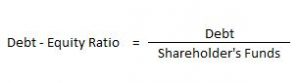Here, Debt includes Long-term Borrowings and Long-term Provisions. Shareholder’s Funds include Share Capital and Reserve & Surplus.

### Solvency Ratio – Total Assets to Debt Ratio

This ratio is a variation of debt-equity ratio. In this ratio, assets are expressed in terms of long term debts.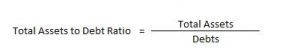Here, Total Assets = Non- Current Assets + Non-Current Investments + Long Term Loans & Advances + Current Assets

Debts = Long-term Borrowings and Long-term Provisions

### Solvency Ratio – Proprietary Ratio

It is the proportion of total assets funded by the shareholders. A higher proprietary ratio is an indicator of sound financial position from long-term point of view.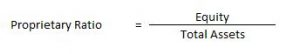### Solvency Ratio – Interest Coverage Ratio

The interest coverage ratio is a debt ratio and profitability ratio used to determine how easily a company can pay interest on its outstanding debt. This ratio indicates how many times the interest charges are covered by the profits available to pay interest charges.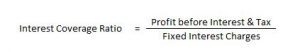### e) Debt to Capital Employed Ratio

This ratio expresses the relation of long-term debt with the sum total of both external and internal funds (i.e., capital employed or net assets).

Debt to capital employed ratio = Long-term Debt/Capital Employed (or Net Assets)

Capital employed can be calculated by taking either the liabilities side or the assets side as the base.

Therefore, it is equal to long-term debt of the company + shareholders’ funds. Or, it can be calculated by deducting current liabilities from the total assets of the enterprise.

The debt to capital employed ratio depicts the proportion of long term debts forming part of the capital employed. Thus, a lower ratio would provide the lenders the security of their funds.

### Solvency Ratio Examples

#### How to compute debt equity ratio – Solvency Ratio Analysis – Question 1

From the following information calculate the debt equity ratio.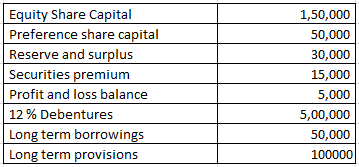#### Explanation : –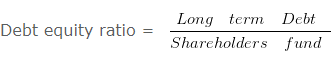= 650000/250000

= 2.6 : 1
Working note 1 : Long term Debt = 12 % Debentures + Long term borrowings + Long term provisions
Long term Debt = 500000 + 50000 + 100000
Long term Debt = 650000
Working note 2 : Shareholders fund = Equity Share Capital + Preference share capital + Reserve and surplus + Securities premium + Profit and loss balance
Shareholders fund = 150000 + 50000 + 30000 + 15000 + 5000
Shareholders fund = 250000

#### When total debts are given – Solvency Ratio Analysis – Question 2

From the following information compute the current ratio.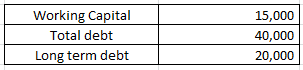#### Explanation : –Current Ratio = 35000/20000

Current Ratio = 1.75 :1
Workings:
Working Capital = Current assets (-) Current liabilities
15000 = Current assets (-) 20000
Current assets = 35000
Workings:
Current liabilities = Total debt (-) Long term debt
Current liabilities = 40000 (-) 20000
Current liabilities = 20000

#### How to compute debt equity ratio – Solvency Ratio Analysis – Question 3

From the following information calculate debt equity ratio.

#### Explanation : –Debt equity ratio = 200000/400000

Debt equity ratio = 0.5 : 1
Working note 1 : Long term Debt = Long term borrowings + Long term provisions
Long term Debt = 120000 + 80000
Long term Debt = 200000
Working note 2 : Shareholders fund = Non current assets + Working capital (-) Non current liabilities
OR
Shareholders fund = Non current assets + Current assets (-) Current liabilities (-) Long term borrowings (-) Long term provisions
Shareholders fund = 500000 + 200000 (-) 100000 (-) 120000 (-) 80000
Shareholders fund = 400000

#### Calculation of Equity ratio – Solvency Ratio Analysis – Question 4

From the Following information calculate Equity Ratio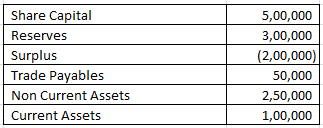#### Explanation : –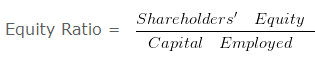Equity Ratio = 600000/300000

Equity Ratio = 2
Working note 1 :
Shareholders’ Equity = Share Capital + Reserves + Surplus
= 500000 + 300000 + -200000
= 600000
Capital employed = Non Current Assets + Current Assets (-) Trade Payables
Capital employed = 250000 + 100000 (-) 50000
Capital employed = 300000

#### Calculation of Proprietary Ratio – Solvency Ratio Analysis – Question 5

Compute Proprietary ratio if equity share capital is Rs. 125000 ,Preference Share Capital is Rs. 100000 ,Capital Reserve is Rs. 80000 ,Profit & Loss Balance is Rs. 55000 . The value of 7 % Debentures is Rs. 62500 and 9 % Mortgage loan- Rs. 112500 .Value of Current Liabilities
is Rs. 262500 Non Current Assets is worth Rs. 275000 Value of Current Assets is Rs. 125000 .

#### Explanation : –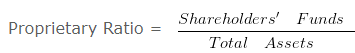Proprietary Ratio = 360000/400000

Proprietary Ratio = 0.9
Working note 1 : Shareholders’ Funds = Equity share capital + Preference share capital + Capital reserve + Profit and loss balance
Shareholders’ Funds = 125000 + 100000 + 80000 + 55000 Shareholders’ Funds = 360000
Working note 2 : Total Assets = Non current assets + Current assets
Total Assets = 275000 + 125000
Total Assets = 400000

#### Debt to total assets ratio – Solvency Ratio Analysis – Question 6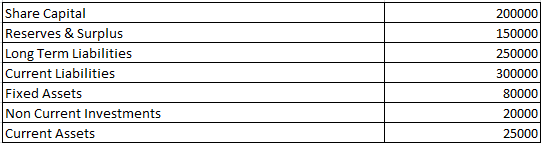Compute Debt to Total Assets Ratio from the above information.

#### Explanation : –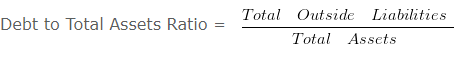Debt to Total Assets Ratio = 250000/125000

Debt to Total Assets Ratio = 2 :1
Working note 1 :
Total Assets = Fixed Assets + Non Current Investments + Current Assets
= 80000 + 20000 + 25000
Total Assets = 125000

#### Interest Coverage Ratio – Solvency Ratio Analysis – Question 7

Compute Interest Coverage ratio if equity share capital is Rs. 1200000 ,Preference Share Capital is Rs. 720000 ,Capital Reserve is Rs. 360000 ,Profit & Loss Balance is Rs. 600000 . The Value of 13 % debentures is Rs. 250000 and 11 % Mortgage loan of Rs. 300000 .The value of Current Liabilities is Rs. 1180000 Non Current Assets is worth Rs. 2400000 Value of Current Assets is Rs. 3000000 .

#### Explanation : –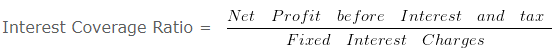Interest Coverage Ratio = 589500/65500

Interest Coverage Ratio = 9 times
Working Notes:
Interest on debenture = 13% x 250000
= 32500
Interest on loan = 11% x 300000
33000
Total interest charges = 6550

#### Interest Coverage Ratio & Debt Service Coverage Ratio – Question 8

Calculate- Interest Coverage Ratio & Debt Service Coverage Ratio from the following information. Net Profit before interest and tax is Rs. 300000 . 5 % Long Term Debt 500000 (Principle amount is repayable in 10 equal installments) .

#### Explanation : –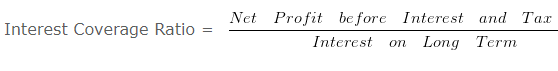= 300000/25000

= 12 times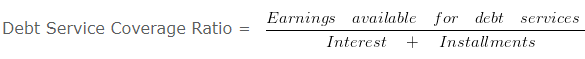Debt Service Coverage Ratio = 300000/(25000+50000)

Debt Service Coverage Ratio = 300000/75000

Debt Service Coverage Ratio = 4 times
Working Notes:
1. Interest on Long Term Debt = 5 % x 500000
25000